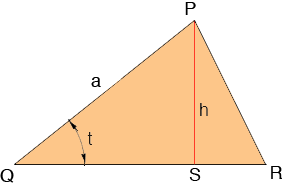SEARCH HOMEMath Central Quandaries & QueriesSubject: Finding the height of a triangle Name: Shanelle Who are you: Student Hey, I'm trying to find the height of a triangle but i only know the base and one of the sides as well as the angle between those two sides...but how can i find the height?Hi Shanelle,In the diagram h is the height so triangle PQS is a right triangle. Thus

sin(t) = h/a

Hence if you know the angle t and the length a you can find h, h = a sin(t).

PennyMath Central is supported by the University of Regina and The Pacific Institute for the Mathematical Sciences.